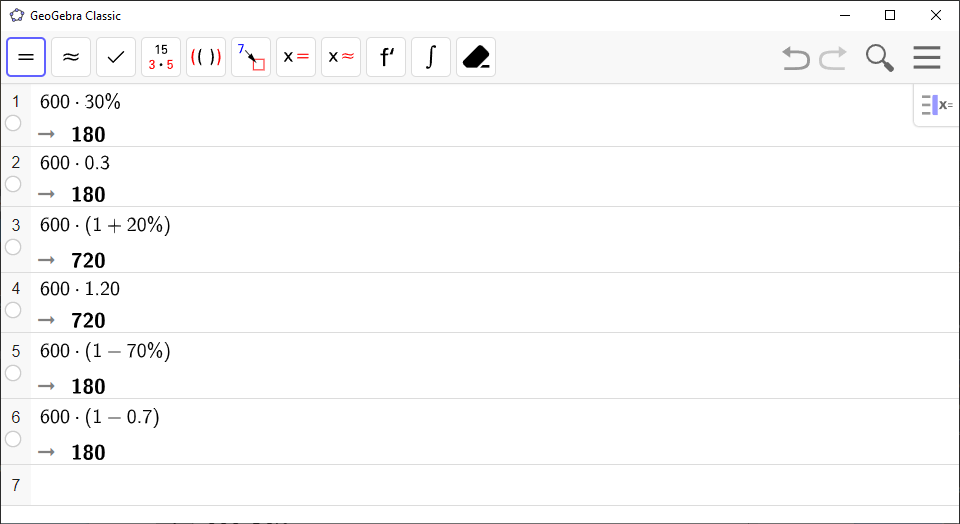# How to Calculate Percent and Growth Factors in GeoGebra

Use `CAS` to compute percentages and growth factors.

In `CAS`, you can calculate percentages directly using the % symbol. If you want to find $30$ % of \$$600$, write: `600*30%`.

A growth factor can be written like this, where $p$ is the percentage: $\left(1±p\phantom{\rule{0.17em}{0ex}}\text{%}\right)$.

`GeoGebra` Instruction 1

1.
Open `CAS` under`View` in`Menu`.
2.
Type your expression into `CAS` using the commands above and press `Enter` or click on `Numeric Evaluation`.